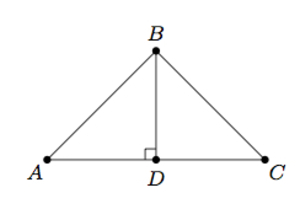# Creating Similar Triangles

Alignments to Content Standards: 8.G.A.4

In triangle $ABC$ below, $\angle B$ is a right angle and $|AB| = |BC|$:Draw a line segment joining one of the vertices of $\triangle ABC$ to the opposite side so that it divides $\triangle ABC$ into two triangles which are both similar to $\triangle ABC$. Explain, using rigid motions and dilations, why the triangles are similar.

## IM Commentary

The purpose of this task is to apply rigid motions and dilations to show that triangles are similar. The teacher will need to monitor students carefully to make sure that they draw an appropriate line segment: for this particular triangle, the only one which will work is the segment from $B$ (the vertex of the right angle) perpendicular to $\overline{AC}$. If students are struggling, the teacher may wish to suggest thinking about the angles of $\triangle ABC$. Since $\angle B$ is a right angle, the two triangles into which $\triangle ABC$ is divided need to be right triangles as well and the only segment that accomplishes this is the one mentioned above. Teachers will also have to provide guidance in terms of what is expected in terms of establishing triangle similarity:

• they may wish to provide patty paper for students to visualize the rigid transformation part of the argument (though students can also argue more abstractly in terms of rigid motions: both forms of argument are provided in the solution).
• while students could use physical measurements or technology to verify the scale factor, it is noteworthy that they have the tools at their disposal to provide an argument based on congruent side lengths (this is supplied as an optional supplement to the solution).

The construction here plays a key role in the high school proof of the Pythagorean Theorem: see https://www.illustrativemathematics.org/illustrations/1568. In this task the students have been given that the right triangle is isosceles but the same argument can be applied in general. Knowing that $\triangle ABC$ is isosceles simplifies the similarity transformation part of the task because students can calculate the angles of the two smaller triangles and conclude that they are also isosceles: the scale factor is then the ratio of leg lengths of $\triangle ABC$ to the leg lengths of the smaller triangles.

## Solution

Since $\triangle ABC$ is a right triangle, for our two smaller triangles to be similar to it, they will also need to be right triangles.  This tells us which vertex our line segment must start from:  If we start from vertex $A$, the line perpendicular to the opposite side is $\overleftrightarrow{AB}$ and this does not divide $\triangle ABC$ into two smaller triangles. Similar reasoning rules out vertex $C$. So our line must start from vertex $B$, and our line segment must be the one starting at $B$ and perpendicular to $\overleftrightarrow{AC}$.  Suppose then that $D$ is the point on $\overline{AC}$ so that $\overleftrightarrow{BD}$ is perpendicular to $\overleftrightarrow{AC}$, shown below:We will first show that $\triangle ADB$ is similar to $\triangle ABC$: the argument for $\triangle CDB$ is much the same. We can translate $D$ to $B$ and then rotate counterclockwise about $B$ so that the right angle $ADB$ matches up with the right angle $CBA$. We can then dilate the rotated triangle about $B$ by a factor of $\frac{|BC|}{|DA|} = \frac{|BA|}{|DB|}$. A detailed explanation of each step in this argument is provided below. While the rigid motion part can be verified with patty paper, showing that $\frac{|BC|}{|DA|} = \frac{|BA|}{|DB|}$ to give us our scale factor, requires a more detailed argument.

We can move vertex $D$ to match up with vertex $B$ by translating along segment $\overline{DB}$. The effect of this is pictured below, with the translated image of $\triangle ADB$ being denoted $\triangle A'D'B'$:Next we apply a rotation, about $B$, through angle $A'BC$. We denote the image of $\triangle A'D'B'$ under the rotation as $\triangle A''D''B''$. The rotation will send $\overrightarrow{D'A'}$ to $\overrightarrow{BC}$ as shown below:We know that $B''$ lies on $\overrightarrow{BA}$ because $\angle B''D''A''$ has the same measure as $\angle ABC$ as both are right angles.

We now apply a dilation with center $B$ which maps $A''$ to $C$ and $B''$ to $A$, which finishes the argument.

(Here is a formal argument that the dilation works as intended:  As long as $\frac{|BC|}{|D''A''|} = \frac{|BA|}{|D''B''|}$, we can take this as our scale factor for the dilation. We know $|BA| = |BC|$ since $\triangle ABC$ is isosceles. We know that $m(\angle A''B''D'') = m(\angle ABD)$ and $m(\angle B''A''D'') = m(\angle BAD)$ since rigid motions do not change angle measures.  But $m(\angle BAD) = 45$ since this is the same as $\angle A$ in $\triangle ABC$. This means that $m(\angle ABD)$ is also 45 because $m(\angle ADB) = 90$ and the sum of the angles in $\triangle ADB$ is 180 degrees.  This means that $|DA| = |DB|$ and so $|D''A''| = |D''B''|$. Since we have already seen that $|BC| = |BA|$ we can conclude that $\frac{|BC|}{|D''A''|} = \frac{|BA|}{|D''B''|}$ and this is the desired scale factor to complete the similarity between $\triangle ADB$ and $\triangle CBA$.)

The similarity between $\triangle CDB$ and $\triangle ABC$ can be shown as above. Alternatively,  $\triangle ABC$ is a right isosceles triangle with line of symmetry $\overleftrightarrow{BD}$. So we can reflect $\triangle CDB$ about $\overleftrightarrow{BD}$, mapping it to $\triangle ADB$. Then we can follow the identical steps outlined above.